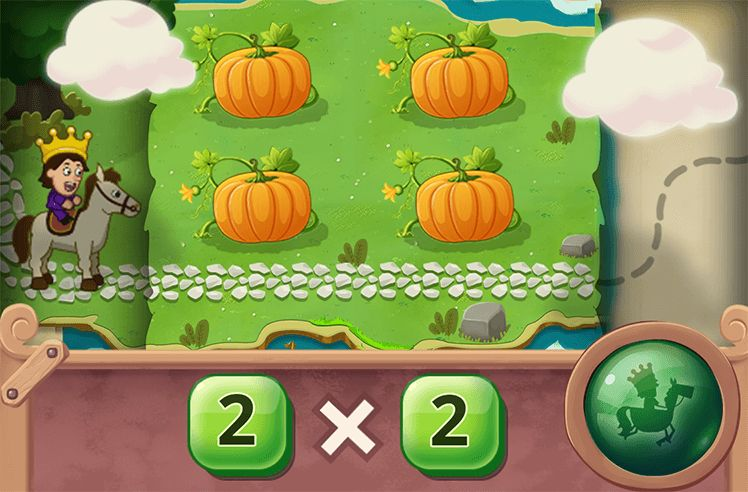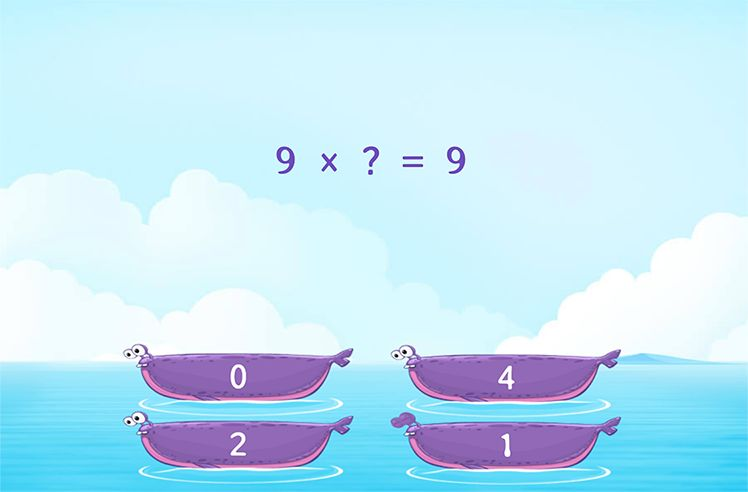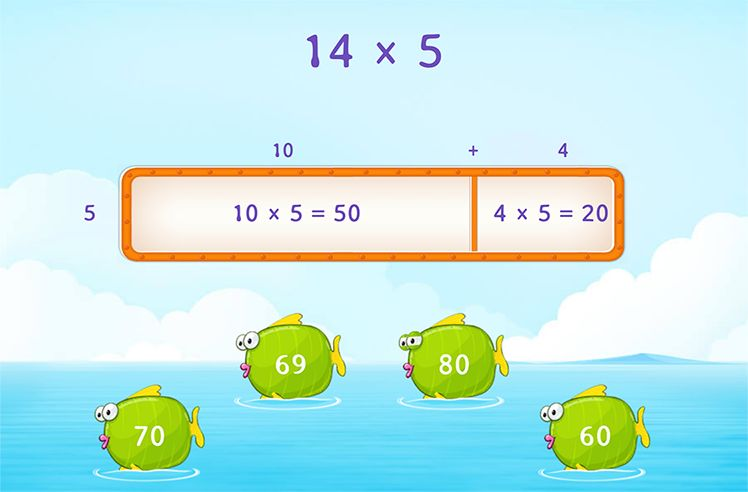# Multiplication Property of Equality – Definition with Examples

» Multiplication Property of Equality – Definition with Examples

## Multiplication Property of Equality

Algebra plays an important role in mathematics. One of the fundamental algebraic concepts states that an equation is a mathematical sentence with an equal sign. We can translate day-to-day activities and transactions to algebraic equations.

Example: 5 + 3 = 8

12 – 7 = 5

Balanced operations of addition, subtraction, multiplication, and division on both sides do not change the truth value of any equation.

The multiplication property of equality states that when we multiply both sides of an equation by the same number, the two sides remain equal.

That is, if ab, and c are real numbers such that a = b, then

a × c = b × c

Example 1 : Lisa and Linda have got the same amount of money. If both of them double their money, that is both of them multiply their money by 2; they still have the same amount of money.

Note that the property holds true even when the multiplicand is zero as zero times any number is zero.

We use this property to solve equations.

Example 2x= 5

Multiply both sides by 4.

x× 4 = 5 × 4

x = 20

To check we can substitute the value of x in the original equation.

20= 5

5 = 5

Example 3: One-fourth of the kids who visited the amusement park “Jump & Slide” on holiday tried their new ride ‘loop-O-loop’. If 75 kids tried the ride, how many kids visited the park that day?

Let ‘a’ be the number of kids who visited the park that day. One-fourth of this number is given to be 75. That is,

a= 75

You need to solve the equation for a.

By the multiplication property of equality, if you multiply both sides by the same number, the equality still holds true. So, multiply both sides by 4.

a4 × 4 = 75 × 4

a = 300

Therefore, the 300 kids visited the park that day.Introduction to Multiplication

Play NowMultiplication Properties

Play Now